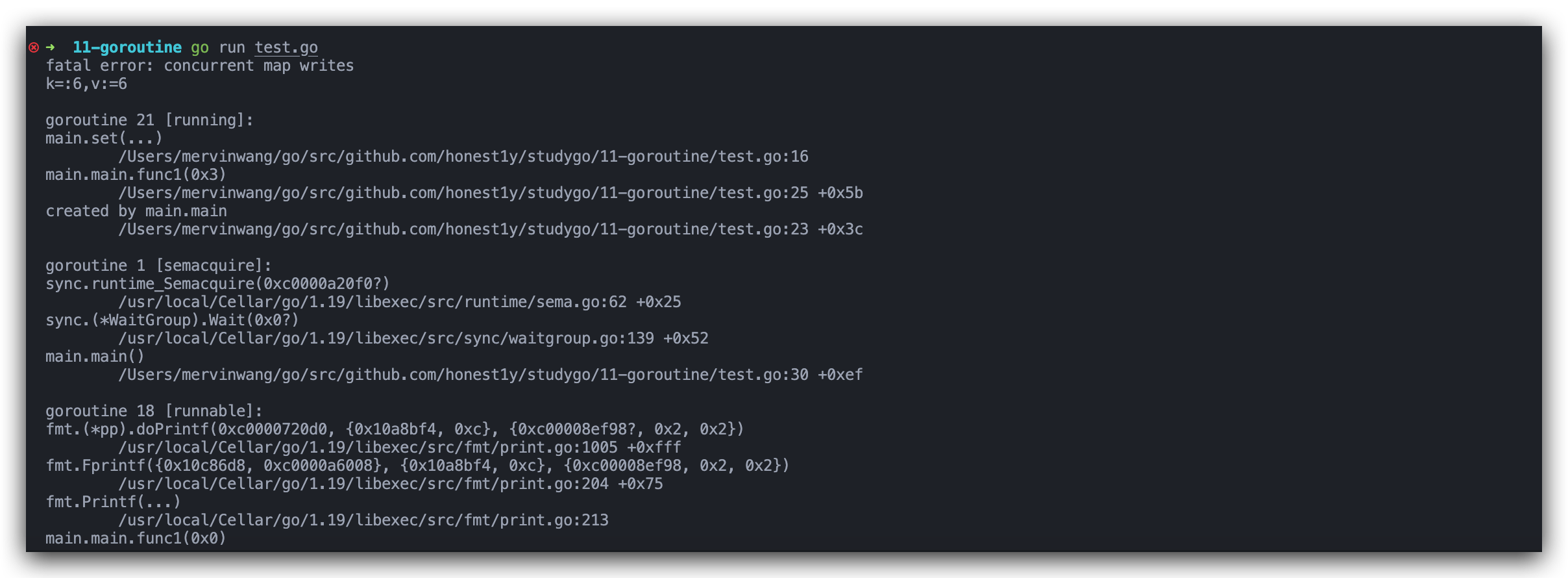# Go语言基础之并发(四) – 并发安全和锁

86次阅读

``````package main

import (
"fmt"
"sync"
)

var (
x int64

wg sync.WaitGroup // 等待组
)

for i := 0; i < 5000; i++ {
x = x + 1
}
wg.Done()
}

func main() {

wg.Wait()
fmt.Println(x)
}``````## 互斥锁

`sync.Mutex`提供了两个方法供我们使用。

func (m *Mutex) Lock() 获取互斥锁
func (m *Mutex) Unlock() 释放互斥锁

``````package main

import (
"fmt"
"sync"
)

// sync.Mutex

var (
x int64

wg sync.WaitGroup // 等待组

m sync.Mutex // 互斥锁
)

for i := 0; i < 5000; i++ {
m.Lock() // 修改x前加锁
x = x + 1
m.Unlock() // 改完解锁
}
wg.Done()
}

func main() {

wg.Wait()
fmt.Println(x)
}``````

## 读写互斥锁

`sync.RWMutex`提供了以下5个方法。

func (rw *RWMutex) Lock() 获取写锁
func (rw *RWMutex) Unlock() 释放写锁
func (rw *RWMutex) RLock() 获取读锁
func (rw *RWMutex) RUnlock() 释放读锁
func (rw *RWMutex) RLocker() Locker 返回一个实现Locker接口的读写锁

• 读锁
• 写锁

``````package main

import (
"fmt"
"sync"
"time"
)

var (
x       int64
wg      sync.WaitGroup
mutex   sync.Mutex
rwMutex sync.RWMutex
)

// writeWithLock 使用互斥锁的写操作
func writeWithLock() {
mutex.Lock() // 加互斥锁
x = x + 1
time.Sleep(10 * time.Millisecond) // 假设写操作耗时10毫秒
mutex.Unlock()                    // 解互斥锁
wg.Done()
}

mutex.Lock()                 // 加互斥锁
time.Sleep(time.Millisecond) // 假设读操作耗时1毫秒
mutex.Unlock()               // 释放互斥锁
wg.Done()
}

// writeWithLock 使用读写互斥锁的写操作
func writeWithRWLock() {
rwMutex.Lock() // 加写锁
x = x + 1
time.Sleep(10 * time.Millisecond) // 假设写操作耗时10毫秒
rwMutex.Unlock()                  // 释放写锁
wg.Done()
}

rwMutex.RLock()              // 加读锁
time.Sleep(time.Millisecond) // 假设读操作耗时1毫秒
rwMutex.RUnlock()            // 释放读锁
wg.Done()
}

func do(wf, rf func(), wc, rc int) {
start := time.Now()
// wc个并发写操作
for i := 0; i < wc; i++ {
go wf()
}

//  rc个并发读操作
for i := 0; i < rc; i++ {
go rf()
}

wg.Wait()
cost := time.Since(start)
fmt.Printf("x:%v cost:%v\n", x, cost)

}

func main() {
do(writeWithLock, readWithLock, 10, 1000) // x:10 cost:1.466500951s
}``````

``````// 使用互斥锁，10并发写，1000并发读
do(writeWithLock, readWithLock, 10, 1000) // x:10 cost:1.466500951s

// 使用读写互斥锁，10并发写，1000并发读
do(writeWithRWLock, readWithRWLock, 10, 1000) // x:10 cost:117.207592ms``````

## sync.WaitGroup

func (wg * WaitGroup) Add(delta int) 计数器+delta
(wg *WaitGroup) Done() 计数器-1
(wg *WaitGroup) Wait() 阻塞直到计数器变为0

`sync.WaitGroup`内部维护着一个计数器，计数器的值可以增加和减少。例如当我们启动了 N 个并发任务时，就将计数器值增加N。每个任务完成时通过调用 Done 方法将计数器减1。通过调用 Wait 来等待并发任务执行完，当计数器值为 0 时，表示所有并发任务已经完成。

``````var wg sync.WaitGroup

func hello() {
defer wg.Done()
fmt.Println("Hello Goroutine!")
}
func main() {
go hello() // 启动另外一个goroutine去执行hello函数
fmt.Println("main goroutine done!")
wg.Wait()
}``````

## sync.Map

Go 语言中内置的 map 不是并发安全的，请看下面这段示例代码。

``````package main

import (
"fmt"
"strconv"
"sync"
)

var m = make(map[string]int)

func get(key string) int {
return m[key]
}

func set(key string, value int) {
m[key] = value
}

func main() {
wg := sync.WaitGroup{}
for i := 0; i < 10; i++ {
go func(n int) {
key := strconv.Itoa(n)
set(key, n)
fmt.Printf("k=:%v,v:=%v\n", key, get(key))
wg.Done()
}(i)
}
wg.Wait()
}``````func (m *Map) Store(key, value interface{}) 存储key-value数据
func (m *Map) Load(key interface{}) (value interface{}, ok bool) 查询key对应的value
func (m *Map) Delete(key interface{}) 删除key
func (m *Map) Range(f func(key, value interface{}) bool) 对map中的每个key-value依次调用f

``````package main

import (
"fmt"
"strconv"
"sync"
)

var m = sync.Map{}

func main() {
wg := sync.WaitGroup{}
for i := 0; i < 20; i++ {
go func(n int) {
key := strconv.Itoa(n)
m.Store(key, n)